Students can Download Tamil Nadu 11th Chemistry Model Question Paper 5 English Medium Pdf, Tamil Nadu 11th Chemistry Model Question Papers helps you to revise the complete Tamilnadu State Board New Syllabus, helps students complete homework assignments and to score high marks in board exams.

## TN State Board 11th Chemistry Model Question Paper 5 English Medium

General Instructions:

1. The question paper comprises of four parts.
2. You are to attempt all the parts. An internal choice of questions is provided wherever applicable.
3. All questions of Part I, II, III and IV are to be attempted separately.
4. Question numbers 1 to 15 in Part I are Multiple Choice Questions of one mark each.
These are to be answered by choosing the most suitable answer from the given four alternatives and writing the option code and the corresponding answer
5. Question numbers 16 to 24 in Part II are two-mark questions. These are to be answered in about one or two sentences.
6. Question numbers 25 to 33 in Part III are three-mark questions. These are to be answered in about three to five short sentences.
7. Question numbers 34 to 38 in Part IV are five-mark questions. These are to be answered in detail Draw diagrams wherever necessary.

Time: 2:30 Hours
Maximum Marks: 70

PART – 1

Answer all the Questions: [15 × 1 = 15]
Choose the most suitable answer from the given four alternatives.

Question 1.
The equivalent mass of ferrous oxalate is …………………….
(a)(b)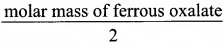(c)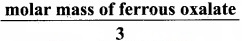(d) None of these
Solution:n = 1 + 2(1) = 3
(c)Question 2.
Time independent Schnodinger wave equation is ………………….
(a) $$\widehat{\mathrm{H}} \psi=\mathrm{E} \psi$$
(b) $$\nabla^{2} \psi+\frac{8 \pi^{2} m}{h^{2}}(E+V) \psi=0$$
(c) $$\frac{\partial^{2} \Psi}{\partial x^{2}}+\frac{\partial^{2} \Psi}{\partial y^{2}}+\frac{\partial^{2} \Psi}{\partial z^{2}}+\frac{2 m}{h^{2}}(E-V) \psi=0$$
(d) All of these
(a) $$\widehat{\mathrm{H}} \psi=\mathrm{E} \psi$$Question 3.
Choose the incorrect statement.
(а) The chemical symbol of nickel is Ni.
(b) An element is a material made up of different kind of atoms.
(c) The physical state of bromine is liquid.
(d) The physical and chemical properties of the elements are periodic functions of their atomic numbers.
(b) An element is a material made up of different kind of atoms.

Question 4.
Assertion: Permanent hardness of water is removed by treatment with washing soda.
Reason: Washing soda reacts with soluble calcium and magnesium chlorides and sulphates in hard water to form insoluble carbonates.
(a) Both assertion and reason are true and reason is the correct explanation of assertion.
(b) Both assertion and reason are true but reason is not the correct explanation of assertion.
(c) Assertion is true but reason is false
(d) Both assertion and reason are false
Solution:
Ca2+ + Na2CO3 → CaCO3↓+ 2Na+
(a) Both assertion and reason are true and reason is the correct explanation of assertion.

Question 5.
Match the following: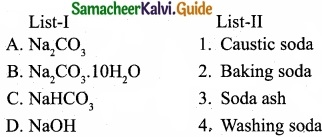Question 6.
Compressibility factor for CO2 at 400 K and 71.0 bar is 0.8697. The molar volume of CO2under these conditions is …………………………
(a) 22.04 dm3
(b) 2.24 dm3
(c) 0.41 dm3
(d) 19.5 dm3
Solution:
Compressibility factor (z) = $$\frac{PV}{nRT}$$
V = $$\frac{z × nRT}{p}$$ = $$\frac{0.8697 \times 1 \times 8.314 \times 10^{-2} \mathrm{bar} \mathrm{dm}^{3} \mathrm{K}^{-1} \mathrm{mol}^{-1} \times 400 \mathrm{K}}{71 \mathrm{bar}}$$
V = 0.41 dm3
(c) 0.41 dm3Question 7.
Thermodynamics is applicable to …………………….
(a) Macroscopic system only
(b) Microscopic system only
(c) Homogenous system only
(d) Heterogeneous system only
(a) Macroscopic system only

Question 8.
In the reaction, Fe(OH)3 (s) ⇄ Fe3+ (aq) + 3OH(aq), if the concentration of OH ions is decreased by $$\frac{1}{4}$$ times, then the equilibrium concentration of Fe3+ will …………………….
(a) Not changed
(b) Also decreased by $$\frac{1}{4}$$ times
(c) Increase by 4 times
(d) Increase by 64 times
Solution: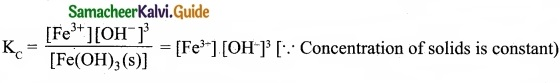When concentration of OH ions declared by $$\frac{1}{4}$$ times, thenTo maintain KC as constant, concentration of Fe3+ will increase by 64 times.
(d) Increase by 64 times

Question 9.
The degree of dissociation a is equal to ……………………
(a) $$\frac{i-1}{n-1}$$
(b) $$\frac{(1-i)n}{n-1}$$
(c) $$\frac{i+1}{n+1}$$
(d) $$\frac{(1+i)n}{n+1}$$
(a) $$\frac{i-1}{n-1}$$Question 10.
The molecules having same hybridisation, shape and number of lone pair of electrons are ………………………..
(a) SeF4, XeO2F2
(b) SF4, XeF2
(c) XeOF4, TeF4
(d) SeCl4, XeF4
Solution:
SeF4, XeO2F2 – sp3d hybridisation
T-shaped, one lone pair on central atom.
(a) SeF4, XeO2F2

Question 11.
Connect pair of compounds which give blue colouration/precipitate and white precipitate respectively, when their Lassaigne’s test is separately done.
(a) NH2NH2HCl and ClCH2 – CHO
(b) NH2CS NH2 and CH3 – CH2Cl
(c) NH2CH2 COOH and NH2 CONH2
(d) C6H5NH2 and ClCH2 – CHO
(d) C6H5NH2 and ClCH2 – CHOQuestion 12.
4-hydroxy phenol oxidised in the presence of K2Cr2O7?H+ to give, …………………….
(a) Quinol
(b) Quinone
(c) Diol
(d) Alkane
(b) Quinone

Question 13.
Methane gas is also called as ……………………
(a) Marsh gas
(b) Mass gas
(c) Molecular gas
(d) Model gas
(a) Marsh gas

Question 14.
The catalyst used in Darzen halogenation of alcohol is ……………………..
(a) CCl4
(b) Acetone
(c) Pyridine
(d) Ethene
(c) PyridineQuestion 15.
Identify the wrong statement in the following.
(a) The clean water would have a BOD value of more than 5 ppm
(b) Greenhouse effect is also called as Global warming
(c) Minute solid particles in air is known as particulate pollutants
(d) Biosphere is the protective blanket of gases surrounding the earth
(a) The clean water would have a BOD value of more than 5 ppm

PART – II

Answer any six questions in which question No. 20 is compulsory. [6 × 2 = 12]

Question 16.
First ionization potential of C-atom is greater than that of B-atom, where as the reverse is true for second ionization potential?
G (Z = 6) 1s2 2s2 2px‘ 2py‘. The electron removal from p orbital is very difficult. So carbon has highest first ionization potential.
B (Z = 5) 1s2 2s2 2p1. In boron nuclear charge is less than that of carbon, so boron has lowest first ionization potential.
I.E1 C > I.E1B

But it is reverse in the case of second ionization energy. Because in case of B+ the electronic configuration is 1s2 2s2, which is completely filled and it has high ionization energy. But in C+ the electronic configuration is 1s2 2s2 2p1, one electron removal is easy so it has low ionization energy.Question 17.
Why H2O2 is used as mild antiseptic?
The oxidising property of hydrogen peroxide and harmless nature of its products such as water and oxygen, leads to oxidation of pollutants in water and act as a mild antiseptic.

Question 18.
An alkali metal (x) forms a hydrated sulphate, X2SO4.10H2O. Is the metal more likely to be sodium (or) potassium?
X forms X2SO4. 10H2O. The metal is more likely be sodium. So X is Na2SO4. 10H2O. It is otherwise called as Glauber’s salt.Question 19.
Define Zeroth law of thermodynamics (or) Law of thermal equilibrium?
Zeroth law of thermodynamics states that ‘If two systems at different temperatures are separately in thermal equilibrium with a third one, then they tend to be in thermal equilibrium with themselves’.

Question 20.
2.82 g of glucose is dissolved in 30 g of water. Calculate the mole fraction of glucose and water?
Solution:
Mass of glucose = 2.82 g
No. of moles of glucose = $$\frac{2.82}{180}$$ = 0.016
Mass of water = 30g = $$\frac{20}{18}$$ = 1.67
xH2O = $$\frac{1.67}{1.67+0.016}$$ = $$\frac{1.67}{1.686}$$ = 0.99
∴xH2O + xxglucose = 1
0.99 + xglucose = 1
xglucose = 1 – 0.99 = 0.01Question 21.
Considering x-axis as molecular axis, which out of the following will form a sigma bond.

1. 1s and 2py
2. 2px and 2px
3. 2px and 2py
4. 1s and 2pz

Along X-axis as molecular axis, only 2px and 2py can form a sigma bond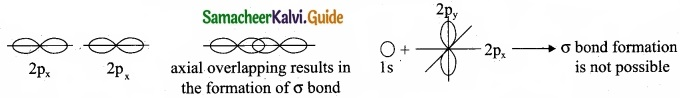2px + 2py and 1s and 2p2 also cannot form σ bond.

Question 22.
What happen when nitrile undergoes acid hydrolysis?
When alkyl nitrile undergoes acid hydrolysis to give amide, which on further hydrolysis to give carboxylic acid.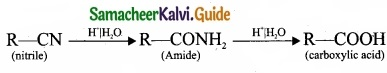Question 23.
How ozone reacts with 2-methyl propene?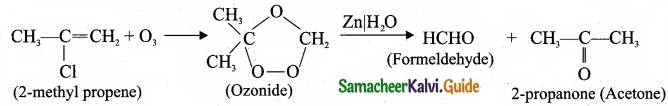Question 24.
What are Freons? Discuss their uses and environmental effects?
Freons are the chlorofluoro derivatives of methane and ethane.
Freon is represented as Freon – cba
Where, c – number of carbon atoms, b = number of hydrogen atoms,
a = total number of fluorine atoms.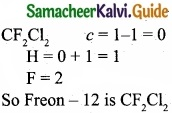Uses of Freons:

1. Freons are used as refrigerants in refrigerators and air conditioners.
2. It is used as a propellant for aerosols and foams.
3. It is used as propellant for foams, to spray out deodorants, shaving creams and insecticides.

Environmental effects of Freons:

1. Freon gas is a very powerful greenhouse gas which means that it traps the heat normally radiated from the earth out into the space. This causes the earth’s temperature to increase, resulting in rising sea levels, droughts, stronger storms, flash floods and a host of other very unpleasant effect. ,

2. As freon moves throughout the air, its chemical ingredients causes depletion of ozone layer. Depletion of ozone increases the amount of ultraviolet radiations that reaches the earths surface, resulting in serious risk to human health. High levels of ozone, in turn, causes respiratory problems and can also kill plants.

PART – III

Answer any six questions in which question No. 26 is compulsory. [6 × 3 = 18]

Question 25.
The first ionisation energy (IE1) and second ionisation energy (IE2) of elements X, Y and Z are given below.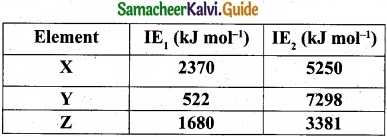Which one of the above elements is the most reactive metal, the least reactive metal and a noble gas?
Noble gases: Ioniation energy ranging from 2372 KJ mol-1 to 1037 kJ mol-1.
For element X, the IE1, value is in the range of noble gas, moreover for this element both IE1 and IE2 are higher and hence X is the noble gas.

For Y, the first ionisation energy is low and second ionisation energy is very high and hence Y is most reactive metal.
For Z, both IE1 and IE2 are higher and hence it is least reactive.Question 26.
An isotope of hydrogen (A) reacts with diatomic molecule of element which occupies group number 16 and period number 2 to give compound (B) is used as a modulator in nuclear reaction. (A) adds on to a compound (C), which has the molecular formula C3H6 to give (D). Identify A, B, C and D?
(I) An isotope of hydrogen Deuterium (A) reacts with diatomic molecule of element belongs to group number 16 and period number 2 oxygen O2 to give a compound (B) which is heavy water D2O. D2O is used as a moderator in nuclear reaction: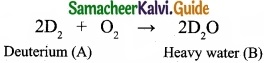(II) Deuterium reacts with C3H6 propane (C) to give Deutero propane C2D6 (D).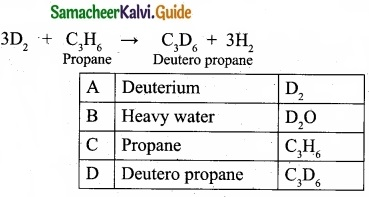Question 27.
Give the uses of gypsum?

1. The Alabaster variety qf gypsum was used by the sculptors.
2. Gypsum is used in making drywalls or plaster boards.
3. Gypsum is used in the production of Plaster of Paris, which is used as a sculpting material.
4. Gypsum is used in making surgical and orthopedic casts.
5. It plays an important role in agriculture as a soil additive, conditioner and fertilizer.
6. Gypsum is used in toothpaste, shampoo and hair products.
7. Calcium sulphate acts as a coagulator in making tofu.
8. It is also used in baking as a dough conditioner.
9. Gypsum is a component of Portland cement, where it acts as a hardening retarder to control the speed at which concrete sets.
10. Gypsum is used to give colour to cosmetics and drugs.
11. Gypsum plays a very important role in wine making.Question 28.
Define inversion temperature?
The temperature below which a gas obey Joule-Thomson effect is called inversion temperature (Ti).
Ti = $$\frac{2a}{2Rb}$$

Question 29.
The vapour pressure of pure benzene (C6H6) at a given temperature is 640 mm Hg. 2.2g , of non-volatile solute is added to 40 g of benzene. The vapour pressure of the solution is 600 mm Hg. Calculate the molar mass of the solute?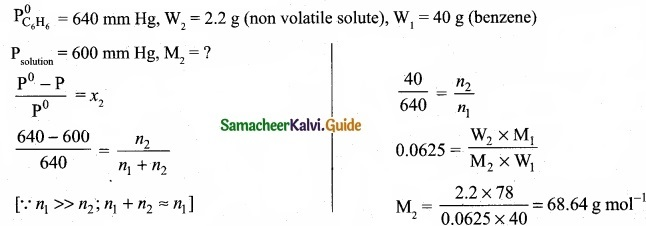Question 30.
In CH4, NH3 and H2O, the central atom undergoes sp3 hybridisation-yet their bond angles are different, why?

1. In CH4, NH3 and H2O the central atom undergoes sp3 hybridisation. But their bond angles are different due to .the presence of lone pair of electrons.
2. It can be explained by VSEPR theory. According to this theory, even though the hybridisation is same, the repulsive force between the bond pairs and lone pairs are not same.
3. Bond pair – Bond pair < Bond pair – Lone pair < Lone pair -Lone pair
So due to the varying repulsive force the bond pairs and lone pairs are distorted from regular geometry and organise themselves in such a way that repulsion will be minimum and stability will be maximum.
4. In case of CH4, there are 4 bond pairs and no lone pair of electrons. So it remains in its regular geometry, i.e., tetrahedral with bond angle = 109° 28’.
5. H2O has 2 bond pairs and 2 lone pairs. There is large repulsion between lp – lp. Again repulsion between lp – bp is more than that of 2 bond pairs. So 2 bonds are more restricted to form inverted V shape (eg;) bent shape molecule with a bond angle of 104° 35.
6. NH3 has 3 bond pairs and 1 lone pair. There is repulsion between lp – bp. So 3 bonds are. more restricted to form pyramidal shape with bond angle equal to 107° 18’.Question 31.
How does hyper conjugation effect explain the stability of alkenes?

1. The relative stability of various classes of carbonium ions may be explained by the number of no bond resonance structures that can be written for them.
2. Such structures are arrived by shifting the bonding electrons from an adjacent C – H bond to the electron deficient carbon.
3. In this way, the positive charge originally on carbon is dispersed to the hydrogen. This manner of electron release by assuming no bond character in the adjacent C – H bond is called hyper conjugation or Baker – Nathan effect.
4. The greater the hyper conjugation, the greater will be the stability of the compound.
The increasing order of stability can be shown as: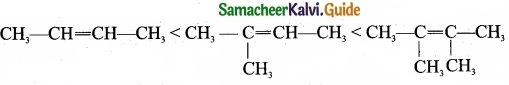5. Alkyl group increases in the C = C double bond carbon, hyper conjugation increases and stability of that organic compound also increases.Question 32.
What is BHC? How will you prepare BHC? Mention its uses?

1. BHC is Benzene hexachloride.

2. Benzene reacts with three molecule of Cl2 in the presence of sunlight or UV light to yield BHC. This is also called as gammaxane or Lindane.3. BHC is a powerful insecticide.Question 33.
Write a chemical reaction useful to prepare the following:

1. Freon-12 from carbon tetrachloride.
2. Carbon tetrachloride from carbon disulphide.

1. Freon – 12 from carbon tetrachloride:
Freon – 12 is prepared by the action of hydrogen fluoride on carbon tetrachloride in the presence of catalytic amount of antimony pentachloride. This reaction is called “Swarts reaction.”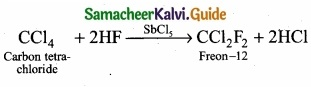2. Carbon tetrachloride from carbon disulphide:
Carbon disulphide reacts with chlorine gas in the presence of anhydrous AlCl3 as catalyst to give carbon tetrachloride.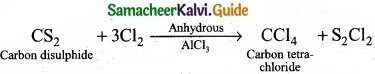PART – IV

Answer all the questions. [5 × 5 = 25]

Question 34 (a).
(I) What is the difference between molecular mass and molar mass? Calculate the molecular mass and molar mass for carbon monoxide.
(II) What are competitive electron transfer reaction? Give example.

[OR]

(b) (I) State the trends in the variation of electronegativity in period and group.
(II) The electron gain enthalpy of chlorine is 348 kJ mol-1. How much energy in kJ is released when 17.5 g of chlorine is completely converted into Cl ions in the gaseous state?
(a) (I) Molecular mass:

1. Relative molecular mass is defined as the ratio of the mass of the molecule to the unified atomic mass unit.
2. It can be calculated by adding the relative atomic masses of its constituent atoms.
3. For carbon monoxide (CO)

Molecular mass = Atomic mass of carbon + Atomic mass of oxygen 12 + 16 = 28 u.

Molar mass:

1. It is defined as the mass of one mole of a substance.
2. The molar mass of a compound is equal to the sum of the relative atomic masses of its constituent expressed in g mol-1.
3. For carbon monoxide (CO)

12 + 16 = 28 g mol-1.

Both molecular mass and molar mass are numerically same but the units are different.

(II) These are the reactions in which redox reactions take place in different vessels and it is an indirect redox reaction. There is a competition for the release of electrons among different metals.

Example:
Zn releases electrons to Cu and Cu releases electrons to Silver and so on.
Zn(s) + Cu2+ → Zn2+(aq) + Cu(s) (Here Zn – oxidised; Cu2+ – reduced)
Cu(s) + 2Ag+ → Cu2+(aq) + 2Ag(s) (Here Cu – oxidised; Ag+ – reduced)

[OR]

(b)
(I) Variation of electronegativity in a period:
The electronegativity increases across a period from left to right. Since the atomic radius decreases in a period, the attraction between the valence electron and the nucleus increases. Hence the tendency to attract shared pair of electrons increases, ‘therefore, electronegativity increases in a period.

(II) Water is amphoteric in nature and it behaves both as an acid as well as base. With acids stronger than itself (e.g., H2S) it behaves as a base and with bases stronger than itself (e.g., NH, ) it acts as an acid.Question 35 (a).
(I) Discuss the three types of Covalent hydrides?
(II) Write the chemical reactions to show the amphoteric nature of water?

[OR]

(b)
(I) Lithium forms monoxide with oxygen whereas sodium forms peroxide with oxygen. Why?
(II) Write about the uses of strontium?
(a) (I)

1. They are the compounds in which hydrogen is attached to another element by sharing of electrons.
2. The most common examples of covalent hydrides are methane, ammonia, waterand hydrogen chloride.
3. Molecular hydrides of hydrogen are further classified into three categories as,
• Electron precise (CH4, C2H6, SiH4, GeH4)
• Electron-deficient (B2H6) and
• Electron-rich hydrides (NH3, H2O)
4. Since most of the covalent hydrides consist of discrete, small molecules that have relatively weak intermolecular forces, they are generally gases or volatile liquids.

(II) Water is amphotenc in nature and it behaves both as an acid as well as base. With acids stronger than itself (e.g., H2S) it behaves as a base and with bases stronger than itself
(e.g., NH3) it acts as an acid.

1. As a base: H2O(l) + H2S(aq) → H3O(aq) – HS(aq)
2. As a acid: H2O(l) + NH3(aq) → OH(aq) + NH+(aq)

[OR]

(b) (I)

1. The fact that a small cation can stabilize a small anion and a large cation can stabilize a large anion explains the formation and stability of the oxides.
2. The size of Li+ ion is very small and it has a strong positive field around it. It can combine . with only small anion, O2- ion, resulting in the formation of monoxide Li2O.
3. The Na+ ion is a larger cation and has a weak positive field around it and can stabilize a bigger peroxide ion, $$\mathrm{O}_{2}^{2-} \text { or }[-\mathrm{O}-\mathrm{O}-]^{2-}$$ resulting in the formation of peroxide Na2O2.

(II)

1. 90Sr is used in cancer therapy.
2. $$^{87} \mathrm{Sr} /^{86} \mathrm{Sr}$$ ratio is used in marine investigators as well as in teeth, tracking animal migrations or in criminal forensics.
3. Dating of rocks.
4. Strontium is used as a radioactive tracer in determining the ‘source of archaeological materials ’ such as timbers and coins.Question 36 (a).
(I) When the driver of an automobile applies brake, the passengers are pushed toward the front of the car but a helium balloon is pushed toward back of the car. Upon forward acceleration the passengers are pushed toward the front of the car. Why?
(II) Critical temperature of H2O, NH3 and CO2 are 647.4, 405.5 and 304.2 K, respectively. When we start cooling from a temperature of 700 K which will liquefy first and which will liquefy finally?

[OR]

(b)
(I) The following water gas shift reaction is an important industrial process for the production of hydrogen gas.
C0(g) + H2O(g) ⇄ CO2(g) + H2(g)
At a given temperature Kp = 2.7. If 0.13 mol of CO, 0.56 mol of water, 0.78 mol of CO2 and 0.28 mol of H2 are introduced into a 2L flask, find out in which direction must the reaction proceed to reach equilibrium.

(II)
2H2O(g) ⇄ 2H2(g) + O2(g) KC = 4.1 × 10-48 At 599 K
N2(g) + O2(g) ⇄ 2NO(g) KC = 1 × 10-30 at 1000 K
Predict the extent of the above two reactions.
(a) (I)
1. When the driver of an automobile applies brake, the passengers are pushed toward the front of the car due to inertia of the body, but a helium balloon pushed toward back of the car. Helium balloon responds to the air around it. Helium molecules are lighter than air of our atmosphere, and so they move toward back by gravity as a result of the accelerating frame.

2. Upon forward acceleration, the passengers are pushed toward the front of the car,because the body in motion tends to stay in motion until acted upon by an outside force. Helium balloon is going to move opposite to this pseudo gravitational force.

(II) Critical temperature of a gas is defined as the temperature above which it cannot be liquefied even at high pressures.
∴ When cooling starts from 700 K, H2O will liquefied first, then followed by ammonia and finally carbon dioxide will liquefied.

[OR]

(b) (I)
CO(g) + H2O(g) ⇄ CO2(g) + H2(g)
Give KP = 2.7
[CO] = 0.13 mol, [H2O] = 0.56 mol
[CO2] = 0.78 mol; [H2] = 0.28 mol
V = 2L
KP = KC (RT)∆ng
2.7 = KC (RT)0
KC = 2.7
QC = $$\frac{\left[\mathrm{CO}_{2}\right]\left[\mathrm{H}_{2}\right]}{[\mathrm{CO}]\left[\mathrm{H}_{2} \mathrm{O}\right]}$$ = $$\frac{\left(\frac{0.78}{2}\right)\left(\frac{0.28}{2}\right)}{\left(\frac{0.13}{2}\right)\left(\frac{0.56}{2}\right)}$$
Q = 3
Q > KC, Hence the reaction proceed in the reverse direction.

(II) In the reactions, decomposition of water at 500 K and oxidation of nitrogen at 1000 K, the value of KC is very less KC < 10-3. So reverse reaction is favoured.
∴ Products << reactantsQuestion 37 (a).
(I) CuCl is more covalent than NaCl. Give reason?
(II) Draw and explain the molecular orbital diagram of Boron molecule?

[OR]

(b)
(I) 0.30 g of a substance gives 0.88 g of carbon dioxide and 0.54 g of water.
Calculate the percentage of carbon and hydrogen in it.

(II) Draw the fisher projection formula for tartaric acid.
(a) (I)
1. Cations having ns2 np6 nd10 configuration show greater polarising power than the cations with ns2 np6 configuration. Hence they show greater covalent character.
2. CuCl is more covalent than NaCl. As compared to Na+ (1.13$$\overset { \circ }{ A }$$), Cu+ (0.6$$\overset { \circ }{ A }$$) is small and has 3s23p63d10 configuration.
3. Electronic configuration of Cu+: [Ar] 3s23p63d10
Electronic configuration of Na+: [He] 2s22p6
So CuCl is more covalent than NaCl.

(II)1. Electronic configuration of B = 1s2 2s2 2p3
2. Electronic configuration of B2 = $$\sigma 1 s^{2} \sigma^{*} 1 s^{2} \sigma 2 s^{2} \sigma^{*} 2 s^{2} \pi 2 p_{x}^{1} \pi 2 p_{z}^{1}$$
3. Bond order = $$\frac { N_{ b }-N_{ a } }{ 2 }$$ = $$\frac{6-4}{2}$$ = 1
4. B2 molecule has two unpaired electrons hence it is paramagnetic.

[OR]

(b) (I) Weight of organic compound = 0.30 g
Weight of carbon-dioxide = 0.88 g
Weight of water = 0.54 g

Percentage of hydrogen:
18 g of water contains 2 g of hydrogen
∴ 0.54 g of water contain = $$\frac{2}{18}$$ × 0.54g of hydrogen
∴% of hydrogen = $$\frac{2}{18}$$ × $$\frac{0.54}{0.30}$$ × 100 = $$\frac{2}{18}$$ × $$\frac{54}{0.3}$$
% of H = 0.111 × 180 = 19.888 ~ 20%

Percentage of carbon:
44 g of water contains 12 g of hydrogen
0.88 g of water contain CO2 contains = $$\frac{12}{44}$$ × 0.88g of hydrogen
∴ % of carbon = $$\frac{12}{44}$$ × $$\frac{0.88}{0.30}$$ × 100 = $$\frac{12}{44}$$ × $$\frac{88}{0.3}$$ = $$\frac{24}{0.3}$$
% of carbon = 80%

(II)Question 38 (a).
An organic compound (A) of a molecular formula C6H6 which is a simple aromatic hydrocarbon. A undergoes hydrogenation to give a cyclic compound (B). A reacts with chlorine in the presence of UV – light to give C which is used as insecticide. Identify A, B and C. Explain the reactions with equation.

[OR]

(b) An organic compound (A) of molecular formula CH2 reacts with methyl magnesium iodide followed by acid hydrolysis to give (B) of molecular formula C2H6O. (B) on reaction with PCl gives (C).(C) on reaction with alcoholic KOH gives (D) an alkene as the product. Identify (A), (B), (C), (D) and explain the reactions involved.

1. Simple aromatic hydrocarbon, C6H6 is benzene.
2. Benzene (A) reacts with H2 in the presence of Pt to give cyclohexane (B).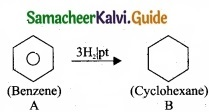3. Benzene (A) reacts with Cl2 in presence of UV-light to give benzene hexachloride (C).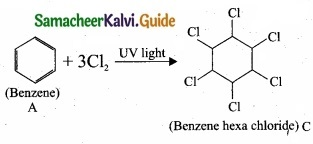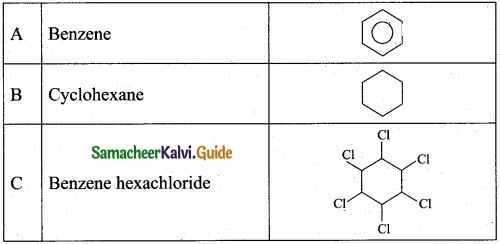[OR]

(b) (I)
1. (A) Of molecular formula CH2O is identified as HCHO, formaldehyde.
2. Formaldehyde reacts with CH3MgI followed by hydrolysis to give ethanol, CH3-CH2OH B as the product.